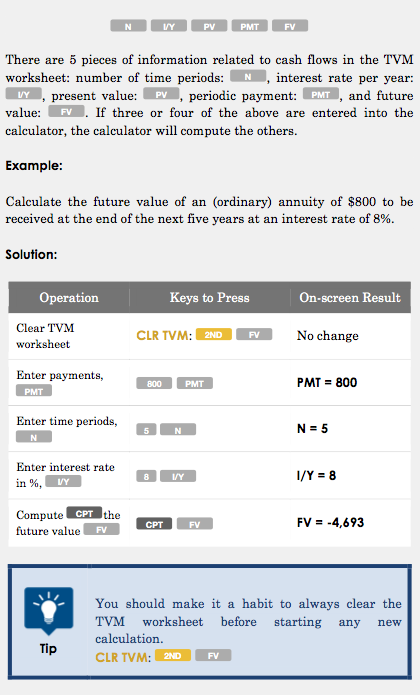CFA CFA Level 1 Present Value calculation – TI BA II Plus Calculator

# Present Value calculation – TI BA II Plus Calculator

• Author
Posts
• 103

Hi
I’m studying for L1 in June and have the below questions

How do you calculate this on the BAII plus calculator. 2(1+g)^5=3. I.e. 2 by 1 plus g to the power of 5 is 3 – calculate g?

Also say if you have a bond that pays \$100 pa for 20 years and the discount rate is 10% is there a fast way to calculate the PV? Or do you actually have to do the calculation out manually or would those type of questions be unlike to appear in the exam?

Thanks very much

Eoghan

• 7

No drama – I’ve worked it out!

• 4

Thanks Artyeasel – much appreciated!

• 2

For 2(1+g)^5=3, I would deduce using algebra, i.e. g = 1-(3/2)^(1/5)
So my keypresses would be [1.5] [Yx] [.2] [=]  [-] [Ans] [=] yielding 1.08.

For the 20 year bond: yes, these questions crop up all the time, and there is a fast way to calculate it. There is already a great example in the BA II Plus guide book that you can get from 300 Hours. I’ll share a screenshot here (@christine let me know if this isn’t cool), but I’d recommend getting it as it probably can help you a lot more: http://www.300hours.com/ba-ii-plus-guide/#.WLfa_RKLTOQ• 2

Sorry in terms of the bond question how would you solve for r the following on the calculator

98 = 10/(1+r) + 10/(1+r)^2 + 110/(1+r)^3

I cant see it in the BA11 manual however it is coming up a lot in my current readings

thanks!